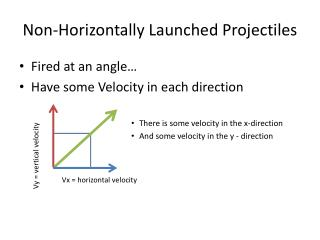DownloadDownload PresentationNon-Horizontally Launched Projectiles

Non-Horizontally Launched Projectiles

Télécharger la présentationNon-Horizontally Launched Projectiles

- - - - - - - - - - - - - - - - - - - - - - - - - - - E N D - - - - - - - - - - - - - - - - - - - - - - - - - - -
Presentation Transcript

1. Non-Horizontally Launched Projectiles • Fired at an angle… • Have some Velocity in each direction • There is some velocity in the x-direction • And some velocity in the y - direction Vy = vertical velocity Vx = horizontal velocity

2. Non-Horizontally Launched Projectiles Shot @ 30 degrees Shot @ 60 degrees Shot closer to vertical So vertical velocity greater than horizontal velocity Vv > Vx • Shot closer to horizontal • So horizontal velocity greater than vertical velocity • Vx > Vy Vy Vy Vx Vx

3. Non-Horizontally Launched Projectiles • Angle for Max Time in the Air? • Time in the air only affected by vertical direction • Launch angle should maximize velocity in the vertical direction • Launch angle for max time in the air – 90 degrees • Vy = maximum amount Vx = 0 !! • All velocity is in the y-direction • No velocity in the x

4. Non-Horizontally Launched Projectiles • Maximum Range – max horizontal displacement • dx must be the biggest number • So we want Vx and time to be the biggest number possible… • But remember… Range, dx = Vx* time of flight

5. Non-Horizontally Launched Projectiles • Maximum Range – max horizontal displacement • dx must be the biggest number • So we want Vx and time to be the biggest number possible… • But remember… • If launch angle is zero – 0 – then… • Vxis at a maximum but time of flight is at a minimum Range, dx = Vx* time of flight

6. Non-Horizontally Launched Projectiles • Maximum Range – max horizontal displacement • dx must be the biggest number • So we want Vx and time to be the biggest number possible… • So which is more important? • Horizontal Vxor vertical time of flight ?Neither!! • Max Range is at 45 degrees!! • Why??? Range, dx = Vx* time of flight

7. Non-Horizontally Launched Projectiles • Maximum Range – max horizontal displacement • At 45 degrees • Vx = Vy • Most of Vx and time Of flight Without sacrificing one for the other Range, dx = Vx* time of flight

8. Non-Horizontally Launched Projectiles • Relationship between 30/60 and 15/75 • 30/60 (and 15/75) have equal displacements because… Range, dx = Vx* time of flight

9. Non-Horizontally Launched Projectiles Shot @ 30 degrees Shot @ 60 degrees Shot closer to vertical Vy Vy • Shot closer to horizontal • Vx of 30 degrees = Vy of 60 degrees • and… Vx of 60 degrees = Vy of 30 degrees… • So… ratio of Vx and time of flight is the same • dx is the same! Vx THEY ARE THE SAME TRIANGLE!!!! Vx